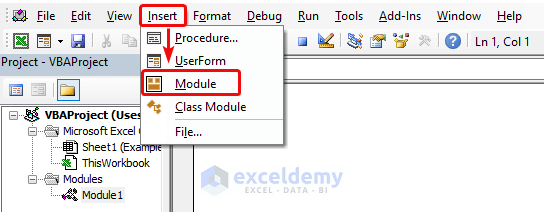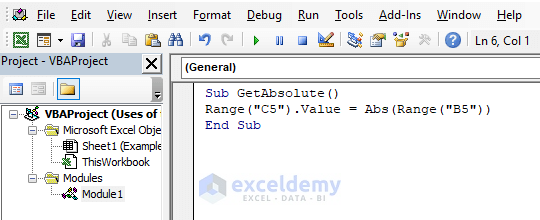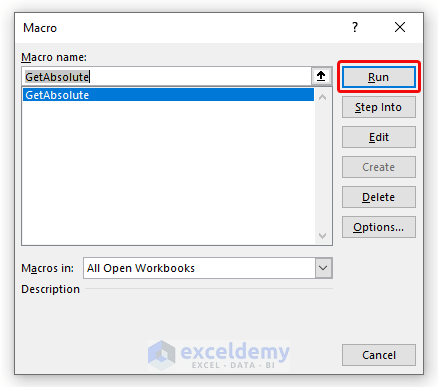# How to Use VBA Abs Function in Excel (9 Examples)

Get FREE Advanced Excel Exercises with Solutions!

The Abs function is a math function in VBA (Visual Basic for Applications) that returns an absolute value for a number. If you insert a negative number into the function, the Abs function will return a positive number. So this function omits the negative sign from a number and returns a positive number. In this article, we will guide you to use the Abs function in Excel VBA with 9 relevant examples.

## 9 Examples of Using Abs Function in Excel VBA

### Example 1: Get the Absolute Value in a Worksheet Cell Using the Abs Function in Excel VBA

In this example, I will show you to get the absolute value for a negative number within an Excel worksheet cell. I have a negative value in cell B5 and using the Abs function in Excel VBA, I will get the absolute value in cell C5.

So let’s get started.

❶ First of all, press the ALT + F11 keys to open the VBA editor.

❷ After that, go to the Insert menu from the main ribbon. Then select Module.

###❸ Now copy the following code and paste it to the VBA editor in Excel.

``````Sub GetAbsolute()

Range("C5").Value = Abs(Range("B5"))

End Sub``````

❹ Save the VBA code using CTRL + S.

###❺ Now press F5 to run the VBA code or press ALT + F8 to open the Macro window.

❻ Select the GetAbsolute function and hit the Run command.

###As a result, you will get the absolute value in cell C5 for the number -5 in cell B5.Read More: How to Use VBA Val Function in Excel (7 Examples)

### Example 2: Input a Number Directly into the Abs Function in Excel VBA

We can input a number directly into the Abs function to get the absolute value of it. To do so, use the following steps.

❶ Open the VBA editor by pressing ALT + F11 keys.

❷ Open a new Module from the Insert menu.

❸ Copy the following code of the Abs function and paste it to the VBA editor.

``````Sub InsertInsideFunction()

MsgBox Abs(-17)

End Sub``````❹ To run the code hit the Run Sub button or press the F5 key.

Finally, you will get the result in a Message Box like this:### Example 3: Get the Absolute Values for a Range of Cells Using the Abs Function Excel VBA

In this section, we will calculate the absolute value for a list of numbers using the Abs function in Excel VBA.

We have a list of numbers in cells B5 to B9. We can get the absolute value for all of them using the Abs function.

To do that,

❶ Press ALT+F11 to open the VBA editor.

❷ Create a new Module from the Insert menu.

❸ Use the following code of the Abs function in the VBA editor.

``````Sub AbsRange()

Dim x As Range

For Each x In Range("B5:B9")

x.Offset(0, 1) = Abs(x.Value)

Next x

End Sub``````

Paste and Save the code in the Excel VBA editor.❺ Press the F5 key.

This will run the code and you will get all the numbers without the negative sign.### Example 4: Get the Absolute Value for a Negative Number in a Message Box Using the Abs Function in Excel VBA

We can insert a negative number in the VBA code and get the absolute value of it with just a keystroke.

To do that follow the steps below.

❶ First of all, press ALT+F11 to open the VBA editor.

❷ Now create a new Module from the Insert menu.

❸ Then use the following code of the Abs function in the VBA editor.

``````Sub AbsMsgBox()

Dim x As Integer

Dim y As Variant

x = -9

y = Abs(x)

MsgBox "Absolute Value of -9 is : " & y

End Sub``````

Paste and Save the code in the Excel VBA editor.❺ Finally hit the F5 key to run the code.

Then you will get the absolute value of -9 is 9 appears in a message box like this:### Example 5: Calculate the Absolute Value for a Positive Number in a Message Box Using the Abs Function in Excel VBA

To calculate the absolute value of a positive number, 144 for instance, follow the steps below:

❶ You can hit ALT+F11 to open the VBA editor.

❷ Go to Insert > Module.

❸ Use the following code of the Abs function in the VBA editor.

``````Sub AbsMsgBox()

Dim x As Integer

Dim y As Variant

x = 144

y = Abs(x)

MsgBox "Absolute Value of 144 is : " & y

End Sub``````

Paste and Save the code in the Excel VBA editor.❺ To run the code, press the F5 key.

Then you will get the absolute value in the following message box.### Example 6: Return an Absolute Value for Zero (0) in a Message Box Using the Abs Function in Excel VBA

To compute the absolute value of zero (0) using the Abs function in Excel VBA, follow the steps below:

❶ To open the VBA editor press ALT+F11.

❷ Now create a new Module from the Insert menu.

❸ Copy the following code of the Abs function in the VBA editor.

``````Sub AbsMsgBox()

Dim x As Integer

Dim y As Variant

x = 0

y = Abs(x)

MsgBox "Absolute Value of 0 is : " & y

End Sub``````

Paste and Save the code in the Excel VBA editor.❺ Finally hit the F5 key to run the above VBA code of the Abs function.

Then you will get the result in the following message box.Related Content: How to Use VBA Space Function in Excel (3 Examples)

### Example 7: Get the Absolute Value of Text String in a Message Box Using the Abs Function in Excel VBA

Let’s see how the Abs function handles a text of string in Excel VBA. To do that, follow the steps below:

❶ Press ALT+F11 to open the VBA editor.

❷ Go to Insert > Module to create a new module for the VBA code.

❸ Copy the following code of the Abs function in the VBA editor.

``````Sub AbsMsgBox()

Dim x As Integer

Dim y As Variant

x = "This is a Text"

y = Abs(x)

MsgBox "Absolute Value of 'This is a Text' is : " & y

End Sub``````

Paste and Save the code in the Excel VBA editor.❺ Press the F5 button to execute the VBA code.

You will see a text message returning “Run-time error ‘13: Type mismatch”. This means the Abs function can’t return an absolute value for a text of string.### Example 8: Return the Absolute Value for a Null Value in a Message Box Using the Abs Function in Excel VBA

To return the absolute value for a null number, follow the steps below:

❶ Hit the ALT+F11 keys to open the VBA editor.

❷ Now create a new Module from the Insert menu.

❸ Copy the following code of the Abs function in the VBA editor.

``````Sub AbsMsgBox()

Dim x

Dim y As Variant

x = Null

y = Abs(x)

MsgBox "Absolute Value of 'Null' is : " & y

End Sub``````

Paste and Save the code in the Excel VBA editor.❺ Now you have to press the F5 key to execute the above VBA code.

After running the code, you will see that the absolute value for a null value is also null.Related Content: How to Use Concatenate in Excel VBA (4 Methods)

### Example 9: Find the Nearest Value to a Number Using the Abs Function in Excel

In this example, I will show you how to find the nearest value to the number 15 using the Abs function in Excel.

We have an array of numbers: 11.3, 43.21, 7.5, 8.44, 7.9, 44.55, 14

So let’s see what number from the above array is the nearest to 15.

To do that,

❶ Press ALT+F11 to open the VBA editor.

❷ Go to Insert > Module to create a new module for the VBA code.

❸ Copy the following code of the Abs function in the VBA editor.

``````Sub FindNearest()

Dim x

Dim y

Dim z As Double

Dim m As Double

Dim n As Double

n = 55

x = Array(11.3, 43.21, 7.5, 8.44, 7.9, 44.55, 14)

For Each y In x

m = Abs(y - 15)

If m < n Then

n = m

z = y

End If

Next y

MsgBox "The value near to 15 is " & z

End Sub``````

Paste and Save the code in the Excel VBA editor.❺ Press the F5 button to execute the VBA code.

After executing the above function, you will see that the nearest number to 15 from the above array is 14.## Things to Remember

• Press ALT + F11 to open the VBA editor.
• Use ALT + F8 keys to open the Macro.
• F5 is the hotkey to run VBA codes.
• To get the absolute value of a number, you have to insert the number or a cell address into the VBA code.

## Conclusion

To sum up, we have discussed 9 relevant examples regarding the usage of the Abs function in Excel. You are recommended to download the practice workbook attached along with this article and practice all the methods with that. And don’t hesitate to ask any questions in the comment section below. We will try to respond to all the relevant queries asap. And please visit our website Exceldemy to explore more.

## Related ArticlesMrinmoy Roy

Hi! I'm Mrinmoy Roy. I'm an Excel and VBA content developer. I write blogs relating to Microsoft Excel on Exceldemy.com. I've completed my graduation in Electronics and Communication Engineering from Khulna University of Engineering & Technology. I've expertise in Excel functions, formulas, Pivot Table, Power Query, Visual Basic, etc. I write blogs to lessen people's hassles while working on Microsoft Excel.

We will be happy to hear your thoughtsAdvanced Excel Exercises with Solutions PDF